RRB NTPC Mock Test - 11 (English)

# RRB NTPC Mock Test - 11 (English)

Test Description

## 100 Questions MCQ Test RRB NTPC Mock Tests Series | RRB NTPC Mock Test - 11 (English)

RRB NTPC Mock Test - 11 (English) for Railways 2023 is part of RRB NTPC Mock Tests Series preparation. The RRB NTPC Mock Test - 11 (English) questions and answers have been prepared according to the Railways exam syllabus.The RRB NTPC Mock Test - 11 (English) MCQs are made for Railways 2023 Exam. Find important definitions, questions, notes, meanings, examples, exercises, MCQs and online tests for RRB NTPC Mock Test - 11 (English) below.
Solutions of RRB NTPC Mock Test - 11 (English) questions in English are available as part of our RRB NTPC Mock Tests Series for Railways & RRB NTPC Mock Test - 11 (English) solutions in Hindi for RRB NTPC Mock Tests Series course. Download more important topics, notes, lectures and mock test series for Railways Exam by signing up for free. Attempt RRB NTPC Mock Test - 11 (English) | 100 questions in 90 minutes | Mock test for Railways preparation | Free important questions MCQ to study RRB NTPC Mock Tests Series for Railways Exam | Download free PDF with solutions
 1 Crore+ students have signed up on EduRev. Have you?
RRB NTPC Mock Test - 11 (English) - Question 1

### If sinθ + cosθ = √3 cos (90 - θ), then what is the value of tanθ?

Detailed Solution for RRB NTPC Mock Test - 11 (English) - Question 1

sinθ + cosθ = √3 cos (90 - θ)

sinθ + cosθ = √3 sinθ

cosθ = √3 sinθ - sinθ

cosθ = sinθ (√3 - 1)

∴ tanθ = 1/(√3 - 1) = (√3 + 1)/2

RRB NTPC Mock Test - 11 (English) - Question 2

### If the difference between the compound interest and the simple interest on a sum of money is 0.0625 times of itself in 2 years. What is the rate of interest?

Detailed Solution for RRB NTPC Mock Test - 11 (English) - Question 2

The formula for CI at annual compound interest, including principal sum, is: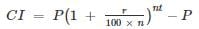Here n = 1

0.0625P = P(1 + r/100)2 - P - (P × r × 2)/100

(1 + r/100)2 - r/50 = 1.0625

r = 25%

RRB NTPC Mock Test - 11 (English) - Question 3

### Where was the capital of Gandhara, one of the sixteen Mahajanpadas?

Detailed Solution for RRB NTPC Mock Test - 11 (English) - Question 3
• Gandhara was an ancient kingdom situated along the Kabul and Swat rivers of Afghanistan and Pakistan.
• The capital of Gandhara was Takshashila also known as Taxila.
• It was one of the sixteen Mahajanpadas of ancient India.
RRB NTPC Mock Test - 11 (English) - Question 4

How many heart chambers are there in a fish?

Detailed Solution for RRB NTPC Mock Test - 11 (English) - Question 4
• Fishes have two heart chambers which consist of a single atrium and a single ventricle.
• The Atrium is responsible for taking the blood from the body. The ventricle is responsible to pump out the blood that has entered the heart.
• Fishes like sharks, bony, trout, tuna, and salmon have two heart chambers.
RRB NTPC Mock Test - 11 (English) - Question 5

Miss Agna lend an amount of Rs. 20,000 divided amongst two borrowers A and B at simple interest rate of 12% and 6% per annum respectively. If the total amount of simple interest earned in 2 years be Rs. 3840, how much amount was lend to A?

Detailed Solution for RRB NTPC Mock Test - 11 (English) - Question 5

Let the amount lend to A be Rs. α = principal amount for A

Amount lend to B = Rs. (20000 – α) = principal amount for B

We know that, Simple interest = (Principal × Rate × Time)/100

Simple interest for A = (α × 12 × 2)/100 = 0.24α

Simple interest for B = [(20000 – α) × 6 × 2]/100 = 2400 – 0.12α

Total interest = simple interest for A + simple interest for B

⇒ 3840 = 0.24α + 2400 – 0.12α

⇒ 0.12α = 3840 – 2400

⇒ α = 1440/0.12 = Rs. 12,000

∴ Amount lend to A = Rs. 12,000

RRB NTPC Mock Test - 11 (English) - Question 6

Find the wrong term in the following series.
BDVHZ, CMOQ, LNP, KO, M.

Detailed Solution for RRB NTPC Mock Test - 11 (English) - Question 6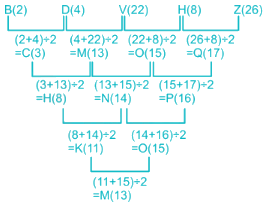Therefore, the right one is HNP instead of LNP.

RRB NTPC Mock Test - 11 (English) - Question 7

In which state will PM Narendra Modi lay the foundation stones for Umiya Dham temple on 5 March 2019?

Detailed Solution for RRB NTPC Mock Test - 11 (English) - Question 7
• PM Narendra Modi is on a 2-day visit to Gujarat from 4 March 2019.
• He will launch the Pradhan Mantri Shram-Yogi Maandhan Yojana (PM-SYM) and inaugurate the first phase of 6-km-long Ahmedabad Metro service.
• He will also lay foundation stones for Umiya Dham temple and launch SAUNI water scheme.
RRB NTPC Mock Test - 11 (English) - Question 8

How many Kirti Chakras were conferred to Armed Forces Personnel by the President in March 2019?

Detailed Solution for RRB NTPC Mock Test - 11 (English) - Question 8
• President Ram Nath Kovind conferred 4 Kirti Chakras and 15 Shaurya Chakras to Armed Forces Personnel on 14 March 2019.
• Major Tushar Gauba was given Kirti Chakra.
• Out of 4, Three Kirti Chakra was given POSTHUMOUSLY

1.SOWAR VIJAY KUMAR

2.RAJENDRA KUMAR NAIN

• Three Kirti Chakras and one Shaurya Chakra were given posthumously.
• Fifteen Param Vishisht Seva Medals, one Uttam Yudh Seva Medals and twenty-five Ati Vishisht Seva Medals to senior officers of the Armed Forces were also conferred.
RRB NTPC Mock Test - 11 (English) - Question 9

A company manufactured a pair of shoes for Rs. 2000 and sold it to a dealer at a profit of 12.5%. The dealer further sold it to a customer at Rs. 2700. What is the profit percentage of the dealer?

Detailed Solution for RRB NTPC Mock Test - 11 (English) - Question 9

Original price of shoes = Rs. 2000

Profit of company = 12.5%

⇒ Price for dealer = 2000 + 12.5% of 2000 = 2000 + 1/8 × 2000 = 2000 + 250 = Rs. 2250

But, selling price of dealer = Rs. 2700

⇒ Profit earned by dealer = 2700 - 2250 = Rs. 450

∴ Profit percentage of dealer = 450/2250 × 100 = 20%

RRB NTPC Mock Test - 11 (English) - Question 10

Uber cup associated with which sport?

Detailed Solution for RRB NTPC Mock Test - 11 (English) - Question 10
• Uber cup associated with Badminton.
• It is named after a former British women's badminton player, Betty Uber.
• It is often called World Team Championships for Women.
• It is a major international badminton competition contested by women's national badminton teams.
• Thomas Cup is the World Team Championship for Men in Badminton.
RRB NTPC Mock Test - 11 (English) - Question 11

In the following figure, rectangle represents Event planners, circle represents Illustrators, triangle represents Game and square represents Indians. Which set of letters represents Event planners who are Games?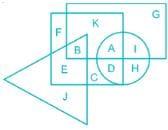Detailed Solution for RRB NTPC Mock Test - 11 (English) - Question 11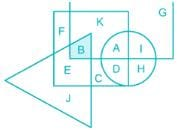The intersection of Rectangle and Triangle represent Event planners who are gamers.

RRB NTPC Mock Test - 11 (English) - Question 12

The following equation is incorrect. Which two signs should be interchanged to correct the equation?
7 × 16 + 20 - 20 ÷ 10 = 31

Detailed Solution for RRB NTPC Mock Test - 11 (English) - Question 12

Interchanging the following signs:

1) ÷ and - → 7 × 16 + 20 ÷ 20 - 10 = 103 ≠ 31

2) × and - → 7 - 16 + 20 × 20 ÷ 10 = 31

3) + and ÷ → 7 × 16 ÷ 20 - 20 + 10 = - 4.4 ≠ 31

4) - and + → 7 × 16 - 20 + 20 ÷ 10 = 94 ≠ 31

Hence, '× and -' is the correct answer.

RRB NTPC Mock Test - 11 (English) - Question 13

What least number must be subtracted from 1936 so that the resulting number when divided by 9, 10 and 15 will leave in case the same remainder 7?

Detailed Solution for RRB NTPC Mock Test - 11 (English) - Question 13

∵ The number should be divisible by 9, 10 and 15 leaving a remainder of 7 in each case.

Thus a remainder of 7 will be also obtained when it is divided by the LCM of 9, 10 and 15.

LCM of 9, 10 and 15 = 90

Dividing 1936 by 90 we get,

Quotient = 21

Remainder = 46

The remainder should be 7

Thus least number which should be subtracted from 1936 = 46 – 7 = 39.

RRB NTPC Mock Test - 11 (English) - Question 14

Point P (-2, 5) is the midpoint of segment AB. Co-ordinates of A are (-5, y) and B are (x, 3). What is the value of x?

Detailed Solution for RRB NTPC Mock Test - 11 (English) - Question 14

We know that the coordinates of mid points of line joining (a1,b1) and (a2,b2) are (a1 + a2)/2 and (b1 + b2)/2

For x coordinate

⇒ -2 = (-5 + x)/2

⇒ -4 = -5 + x

∴ x = 5 - 4 = 1

RRB NTPC Mock Test - 11 (English) - Question 15

The roots of the equation ax2 + x + b = 0 are equal if

Detailed Solution for RRB NTPC Mock Test - 11 (English) - Question 15

The roots of a quadratic equation px2 + qx + r = 0 are equal only when q2 = 4pr

Given equation,

ax2 + x + b = 0

By comparing the above equation with px2 + qx + r = 0, we get

p = a;

q = 1

r = b

Thus putting the values in q2 = 4pr, we get

1 = 4ab

⇒ ab = 1/4

RRB NTPC Mock Test - 11 (English) - Question 16

The world's largest temple is located in which of the following country?

Detailed Solution for RRB NTPC Mock Test - 11 (English) - Question 16

Angkor Wat, also known as the 'city temple', is the largest temple in the world.

• It has a vast temple complex in Cambodia featuring the magnificent remains of several capitals of the Khmer Empire, from the 9th to the 15th century AD.

• It stands on a raised terrace above the rest of the city.

• The temple is made of three rectangular galleries rising to a central tower, each level higher than the last.

RRB NTPC Mock Test - 11 (English) - Question 17

If ‘A’ denotes ‘÷’, ‘B’ denotes ‘×’, ‘C’ denotes ‘+’, ‘D’ denotes’- ‘, then find the value of
38 C 12 B 4 A 2 C 5 A 5?

Detailed Solution for RRB NTPC Mock Test - 11 (English) - Question 17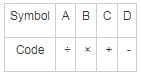Given: 38 C 12 B 4 A 2 C 5 A 5

Substituting the symbols:

38 + 12 × 4 ÷ 2 + 5 ÷ 5

Solution through BODMAS:

38 + 12 × 4 ÷ 2 + 5 ÷ 5

38 + 24 + 1 = 63

Hence, the correct answer is 63.

RRB NTPC Mock Test - 11 (English) - Question 18

Find the variance of the given numbers: 36, 28, 45, and 51.

Detailed Solution for RRB NTPC Mock Test - 11 (English) - Question 18

Mean is the average of the given numbers,

⇒ Mean = (36 + 28 + 45 + 51)/4 = 160/4 = 40

Variance is calculated by taking the average of the squares of the difference between each term and the mean,

⇒ Variance = [(36 - 40)2 + (28 - 40)2 + (45 - 40)2 + (51 - 40)2]/4

= [16 + 144 + 25 + 121]/4 = 306/4 = 76.5

∴ Variance of the given numbers = 76.5

RRB NTPC Mock Test - 11 (English) - Question 19

Asad Ali Khan is associated with which among the following musical instruments?

Detailed Solution for RRB NTPC Mock Test - 11 (English) - Question 19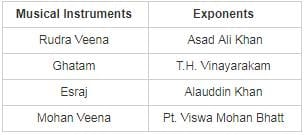RRB NTPC Mock Test - 11 (English) - Question 20

Where is the Arakan Yoma (a range of the Himalaya) located?

Detailed Solution for RRB NTPC Mock Test - 11 (English) - Question 20

The Arakan Mountains, also known as the Rakhine Mountains, are a mountain range in western Myanmar, between the coast of Rakhine State and the Central Myanmar Basin, in which flows the Irrawaddy River.

• It is the most prominent of a series of parallel ridges that arc through Assam, Nagaland, Mizoram and Myanmar.

Hence, 2 is the correct answer.

RRB NTPC Mock Test - 11 (English) - Question 21

Avogadro’s Law shows a relationship between ________.

Detailed Solution for RRB NTPC Mock Test - 11 (English) - Question 21

Avogadro’s Law states that at constant temperature and pressure, all equal volume of gases will have an equal number of molecules" which means 'V' is directly proportional to the 'Number of molecules'.

RRB NTPC Mock Test - 11 (English) - Question 22

In the following question, select the related letters / number from the given alternatives.
N × V : 14 × 22 as Z × F : ?

Detailed Solution for RRB NTPC Mock Test - 11 (English) - Question 22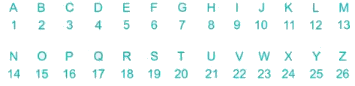Here the numbers denotes the place value of letter in English alphabetical series.

N → 14

V → 22

Z → 26

F → 6

Hence Z × F is 26 × 6.

RRB NTPC Mock Test - 11 (English) - Question 23

Who was the founder of the Satavahana Empire?

Detailed Solution for RRB NTPC Mock Test - 11 (English) - Question 23

Simuka was an Indian king belonging to the Satavahana dynasty. He is mentioned as the first king in a list of royals in a Satavahana inscription at Nanaghat

RRB NTPC Mock Test - 11 (English) - Question 24

Shyam spends a part of his monthly income to the rent and other for savings, the ratio of his expenditure to his savings is 21 : 4. If his monthly income is Rs. 9475, what is the amount of his monthly savings?

Detailed Solution for RRB NTPC Mock Test - 11 (English) - Question 24

Expenditure/savings = 21/4 = 21x : 4x

Income = expenditure + savings

9475 = 21x + 4x

9475 = 25x

x = 379.

∴ Monthly savings = 4x = 4 × 379 = Rs. 1516

RRB NTPC Mock Test - 11 (English) - Question 25

Diameter of wheel of a cycle is 21 cm. The cyclist takes 45 minutes to reach a destination at a speed of 16.5 km/hr. How many revolutions will the wheel make during the journey?

Detailed Solution for RRB NTPC Mock Test - 11 (English) - Question 25

Perimeter of tyre can be given as = πD = (22/7) × 21

⇒ Perimeter of tyre = 66 cm = .00066 km

Distance covered by cyclist in 45 minutes or 45/60 hrs can be given as

⇒ 16.5 × (45/60)

⇒ 16.5 × 3/4

Revolution can be given as total distance covered by cycle/perimeter of tyre

⇒ 16.5 × 3/(4 × .00066) = 18750

∴ The total revolutions are 18750

RRB NTPC Mock Test - 11 (English) - Question 26

The average of 6 consecutive even numbers is 25. If the next even number is also considered, then what is the new average?

Detailed Solution for RRB NTPC Mock Test - 11 (English) - Question 26

Let the first integer be 2x.

Average of 6 consecutive integers: (2x + (2x + 2) + ... + (2x + 10))/6= 12x + 2(1 + 2 + ... + 5) = 12x + 30 = 25 × 6 = 150

⇒ x = 10.

Average of new numbers: (2x + (2x + 2) + ... + (2x + 12) )/ 7 = (14x + 42)/7 = 2x + 6 = 20 + 6 = 26.

RRB NTPC Mock Test - 11 (English) - Question 27

Pipe A can fill a tank in 12 hours and Pipe B can fill the tank in 18 hours. If both the pipes are opened on alternate hours and if pipe B is opened first, then in how much time (in hours) the tank will be full?

Detailed Solution for RRB NTPC Mock Test - 11 (English) - Question 27

1-hour Efficiency of pipe A: 1/12

1-hour Efficiency of pipe B: 1/18

If both opened together, work done in 2 hours: 1/18 + 1/12 = 5/36

After 14 hours, work done = 5 × 7/36 = 35/36.

Work left = 1 – 35/36 = 1/36. This work will be done by pipe B in h hours.

⇒ h/18 = 1/36

⇒ h = 1/2

Therefore, total time = 14.5 hours

RRB NTPC Mock Test - 11 (English) - Question 28

During photosynthesis the oxygen in glucose comes from ______.

Detailed Solution for RRB NTPC Mock Test - 11 (English) - Question 28
• Photosynthesis is the process by which plants, some bacteria and some protistans use the energy from sunlight to produce glucose from carbon dioxide.
• This glucose can be converted into pyruvate which releases adenosine triphosphate (ATP) by cellular respiration. Oxygen is also formed.
RRB NTPC Mock Test - 11 (English) - Question 29

A, B and C can do a job working alone in 6, 9 and 18 days respectively. They all work together for 1 day, then A and B quit. How many days C working alone will take to complete the remainder of the job?

Detailed Solution for RRB NTPC Mock Test - 11 (English) - Question 29

A’s 1 day work = 1/6

B’s 1 day work = 1/9

C’s 1 day work = 1/18

Let C alone worked for ‘x’ days

∵ A and B worked only for one day and C worked for (x + 1) days,

⇒ A’s 1 day work + B’s 1 day work + C’s (x + 1) days’ work = 1

⇒ 1/6 + 1/9 + (x + 1)/18 = 1

⇒ (x + 1)/18 = 13/18

⇒ x + 1 = 13

⇒ x = 13 – 1 = 12

∴ C worked alone for 12 days

RRB NTPC Mock Test - 11 (English) - Question 30

What is the simplified value of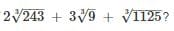Detailed Solution for RRB NTPC Mock Test - 11 (English) - Question 30

Given that,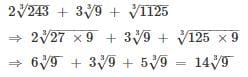RRB NTPC Mock Test - 11 (English) - Question 31

Who is the author of book named “An Area of darkness”?

Detailed Solution for RRB NTPC Mock Test - 11 (English) - Question 31

V. S. Naipaul is the author of the book named “An Area of darkness”.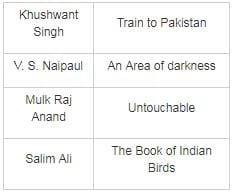RRB NTPC Mock Test - 11 (English) - Question 32

Find out which of the figures (1), (2), (3) and (4) can be formed from the pieces given in figure X? (using all pieces only once)Detailed Solution for RRB NTPC Mock Test - 11 (English) - Question 32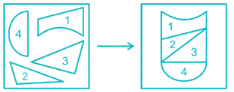Hence, the below figure can be formed from the pieces given in the figure (X).RRB NTPC Mock Test - 11 (English) - Question 33

In the following question, select the one which is different from the other three responses.

Detailed Solution for RRB NTPC Mock Test - 11 (English) - Question 33

If we give number to each letter according to its alphabetical order we find that,

B + D = F i.e. 2 + 4 = 6

H + K = S i.e. 8 + 11 = 19

A + X = Y i.e. 1 + 24 = 25

M + B = 13 + 2 ≠ 17 (Q)

Therefore, MBQ will be the answer.

RRB NTPC Mock Test - 11 (English) - Question 34

In the following question, select the missing number from the given series.
25, 33, 42, 52, 63, ?

Detailed Solution for RRB NTPC Mock Test - 11 (English) - Question 34

25 + 8 = 33

33 + 9 = 42

42 + 10 = 52

52 + 11 = 63

63 + 12 = 75

Hence, 75 is the correct answer.

RRB NTPC Mock Test - 11 (English) - Question 35

Butyric acid is found in________.

Detailed Solution for RRB NTPC Mock Test - 11 (English) - Question 35

• Butyric acid is a short-chain fatty acid found in Rancid butter. It is also found in milk and parmesan cheese.

• The acid is an oily, colorless liquid that is easily soluble in water, ethanol, and ether. It has an unpleasant smell and acrid taste.

• The structural formula of Butyric acid CH3CH2CH2-CO-OH.

• Due to its powerful odor, it is used as a fishing bait additive.

RRB NTPC Mock Test - 11 (English) - Question 36

The volume of a conical tent is 154 cm3 and the area of its base is 38.5 cm2. What is the length (in cm) of canvas required to build the tent, if the canvas is 2 cm in width?

Detailed Solution for RRB NTPC Mock Test - 11 (English) - Question 36

Radius of tent = r and area of its base is 38.5 cm2 = πr2

Then r = √(12.25) = 3.5

Also conical volume = 1/3 πr2h=154

h = 12 and l = 12.5

Curved surface area = πrl = 137.50 cm2

Curved surface area of conical tent = Area of canvas = length × width = 137.50 cm2

Length = 137.50/width = 137.37/2 = 68.75

RRB NTPC Mock Test - 11 (English) - Question 37

Select the number which can be placed in the sign of question mark (?) from the given alternatives.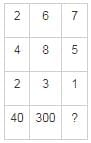Detailed Solution for RRB NTPC Mock Test - 11 (English) - Question 37

The pattern followed here is,

(22 + 42) × 2 = 40;

(62 + 82) × 3 = 300;

Similarly,

(72 + 52) × 1 = 74

Hence, 74 is the correct answer.

RRB NTPC Mock Test - 11 (English) - Question 38

From the given alternative words select the word which cannot be formed using the letters of the given word ‘EXTRAJUDICIAL’?

Detailed Solution for RRB NTPC Mock Test - 11 (English) - Question 38

DIMER → EXTRAJUDICIAL → There is no letter ‘M’ so it cannot be formed.

TRACE → EXTRAJUDICIAL → can be formed.

RACIAL → EXTRAJUDICIAL → can be formed.

TRAIL → EXTRAJUDICIAL → can be formed.

Hence, " DIMER " is the answer.

RRB NTPC Mock Test - 11 (English) - Question 39

A boy added all natural numbers from 1 to 12, however he added one number twice due to which the sum becomes 80. What is the number which he added twice?

Detailed Solution for RRB NTPC Mock Test - 11 (English) - Question 39

Sum of all natural numbers from 1 to 12 = 78

By adding a number twice sum becomes 80

∴ the number which is added twice = 80 - 78 = 2

RRB NTPC Mock Test - 11 (English) - Question 40

Which state has become the first state in India to have a special dog squad for detecting liquor consignment?

Detailed Solution for RRB NTPC Mock Test - 11 (English) - Question 40
• Bihar has become the first state in India to have a special dog squad for detecting liquor consignment.
• The state also imposed a complete ban on liquor 2 years ago.
• 20 trained dogs are a part of the squad at present and were provided 9 months of intense training in Hyderabad.
• The dog squads are handled by Crime Investigation Department (CID).
RRB NTPC Mock Test - 11 (English) - Question 41

Glycogen, starch and cellulose are polymers of

Detailed Solution for RRB NTPC Mock Test - 11 (English) - Question 41
• Glycogen, starch and cellulose are polymers of Glucose.
• Polymers are materials made of long, repeating chains of molecules.
• Glycogen serves as a form of energy storage in humans, animals, fungi, and bacteria.
• Starch is a polymer made by plants to store energy.
• Cellulose is the main substance that makes up the cell walls and fibers of plants.
RRB NTPC Mock Test - 11 (English) - Question 42

Which of the following is not an operating system in any computer?

Detailed Solution for RRB NTPC Mock Test - 11 (English) - Question 42
• Pentium is the brand name of a series of microprocessors produced by Intel Corporation.
• The first microprocessor in the Pentium series was the Pentium 1.
RRB NTPC Mock Test - 11 (English) - Question 43

In the following figure, rectangle represents actors, circle represents illustrator, triangle represents sculptors and square represents gymnasts. Which set of letters represents actors who are sculptors?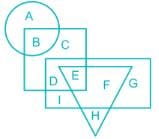Detailed Solution for RRB NTPC Mock Test - 11 (English) - Question 43

Rectangle represents actors,

Circle represents illustrator,

Triangle represents sculptors,

Square represents gymnasts

Intersection of rectangle and triangle represents actors who are sculptors : EF

Hence, EF is the correct answer.

RRB NTPC Mock Test - 11 (English) - Question 44

In the following questions, in four out of the given five pairs of figures, figure I is related to figure II in the same particular manner. Find out the pair in which this relationship does not exist between figure I and II.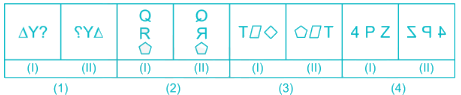Detailed Solution for RRB NTPC Mock Test - 11 (English) - Question 44

In each pair of I and II figure the II figure is a mirror image of figure I.

In the figure given below, the figure II is not the mirror image of figure I.RRB NTPC Mock Test - 11 (English) - Question 45

The shadow of the pole increases by 10 metre when the latitude of sun changes from 45° to 30°, then the height of the pole is?

Detailed Solution for RRB NTPC Mock Test - 11 (English) - Question 45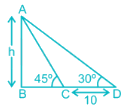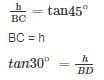BD = h√3

BD = BC + CD

BD = h + 10

h + 10 = h√3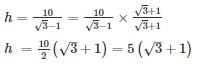h = 5 × 2.732

h = 13.7

RRB NTPC Mock Test - 11 (English) - Question 46

Which city is known as the City of Pearls?

Detailed Solution for RRB NTPC Mock Test - 11 (English) - Question 46

Hyderabad city of Telangana is known as the City of Pearls as the city deals in pearls and diamonds. Hyderabad is also known as Hi-Tech City and City of Nizams.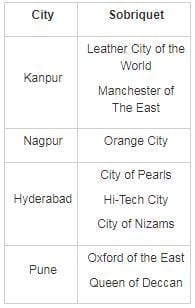RRB NTPC Mock Test - 11 (English) - Question 47

The difference between the ages of two sisters is 2 years when father’s age was 52. Father is elder by 2 years to mother. Elder sister’s age is half of mother’s age. Find the age of younger sister?

Detailed Solution for RRB NTPC Mock Test - 11 (English) - Question 47

Let the elder sister's age be x and younger sister's age be y

Given that difference between two the ages of to sisters is 2 years

⇒ x - y = 2

Given that father's age is 52, then mother's age = 50

Also given that elder sister's age x = half of the mother's age = 50/2 = 25

Substitute x = 25 in x - y = 2

⇒ 25 - y = 2

⇒ y = 25 - 2 = 23

RRB NTPC Mock Test - 11 (English) - Question 48

In Indian Constitution “The Comptroller and Audit General of India” was placed under?

Detailed Solution for RRB NTPC Mock Test - 11 (English) - Question 48

In Indian Constitution “The Comptroller and Audit General of India” was placed under the section of Finance, Accounts, and Audit of Part V and Chapter V.

RRB NTPC Mock Test - 11 (English) - Question 49

Which of the following diseases is caused by viruses?

Detailed Solution for RRB NTPC Mock Test - 11 (English) - Question 49
• Chicken Pox is caused by Varicella Zoster Virus (VZV).
• The disease results in skin rash that forms small, itchy blisters on skin.
• Chickenpox is an airborne disease which spreads easily through the coughs and sneezes of an infected person.
RRB NTPC Mock Test - 11 (English) - Question 50

Rajat is the brother of Sanjay and Manish is the father of Rajat. Jay is the brother of Shreya who is the daughter of Sanjay. Who is the uncle of Jay.

Detailed Solution for RRB NTPC Mock Test - 11 (English) - Question 50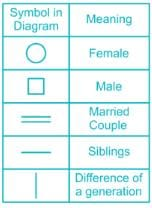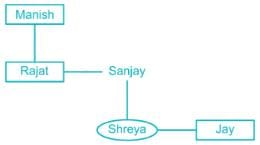Hence, Rajat is the uncle of Jay.

RRB NTPC Mock Test - 11 (English) - Question 51

Tawa River is the tributary of which of the following river?

Detailed Solution for RRB NTPC Mock Test - 11 (English) - Question 51

Tawa River is the tributary of Narmada river. It is the longest tributary of Narmada river. Its origin is in the Satpura range. It meets the Narmada at Hoshangabad district. Its length is 172 km.

RRB NTPC Mock Test - 11 (English) - Question 52

How many Fundamental Duties have been set out in Part-IV A of our Constitution?

Detailed Solution for RRB NTPC Mock Test - 11 (English) - Question 52

11 Fundamental Duties have been set out in Part-IV A of our Constitution. These were added by the 42nd and 86th Amendment Acts in the Constitution.

RRB NTPC Mock Test - 11 (English) - Question 53

Helsinki is the capital of ________.

Detailed Solution for RRB NTPC Mock Test - 11 (English) - Question 53

Helsinki is the capital and largest city of Finland.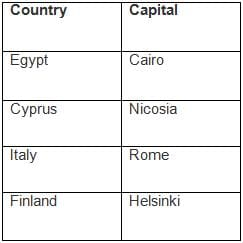RRB NTPC Mock Test - 11 (English) - Question 54

A vendor buys bananas at 4 for Rs. 3 and sells at 3 for Rs. 4. What will be the result?

Detailed Solution for RRB NTPC Mock Test - 11 (English) - Question 54

Vendor buys bananas at 4 for Rs 3 ⇒ cost price of 1 banana = Rs. 3/4

Vendor sells bananas at 3 for Rs 4 ⇒ selling price of 1 banana = Rs. 4/3

We see that selling price is more than the cost price which implies there is a profit.

Profit % = [(4/3 - 3/4)/3/4] × 100 = 77.7%

∴ There is profit of 77.7%

RRB NTPC Mock Test - 11 (English) - Question 55

Who among the following won the gold medal at the 38th GeeBee Boxing Tournament in Helsinki, Finland on 10 March 2019?

Detailed Solution for RRB NTPC Mock Test - 11 (English) - Question 55
• Kavinder Singh Bisht (56kg) struck gold at the 38th GeeBee Boxing Tournament in Helsinki, Finland on 10 March 2019.
• Shiva Thapa (60kg), Govind Sahani (49kg), Mohammed Hussamuddin (56kg) and Dinesh Dagar (69kg) claimed silver medals in the event.
• Sumit Sangwan (91kg), Sachin Siwach (52kg) and Naveen Kumar (+91kg) claimed bronze medals.
RRB NTPC Mock Test - 11 (English) - Question 56

Directions: In the question below is given a statement followed by two assumptions numbered I and II. An assumption is something supposed or taken for granted. You have to consider the statement and the following assumptions and decide which of the assumptions is implicit in the statement.

Statement: If you want to give good results in the limited time period, work smart rather than working hard. It is bound to work- A said to B.

Assumptions:
I. B listens to A.
II. Smart work helps in giving good results in a limited time period.

Detailed Solution for RRB NTPC Mock Test - 11 (English) - Question 56

Statement II is implicit as it is bound to work as told by A. A advises B but this does not mean that we can assume that the latter listens to his/ her advice. Thus, only assumption II is implicit.

RRB NTPC Mock Test - 11 (English) - Question 57

The ratio of number of boys and girls in a school is 3 : 2.If 20% of the boys and 30% of the Girls are scholarship holders, then the percentage of students, who do not get Scholarship, is

Detailed Solution for RRB NTPC Mock Test - 11 (English) - Question 57

Let the number of boys

= 3x and that of girls = 2x

Number of boys who do not hold scholarship

= 80% of 3x =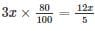Number of girls who do not hold scholarship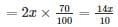Number of students who do not hold scholarship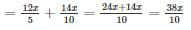Required percentage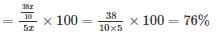RRB NTPC Mock Test - 11 (English) - Question 58

A word is represented by only one set of numbers as given in any one of the alternatives. The sets of number given in the alternatives are represented by two classes of alphabets as shown in the given two matrices. The columns and rows of Matrix-I are numbered from 0 to 4 and that of Matrix-II are numbered from 5 to 9. A letter from these matrices can be represented first by its row and next by its column, for example, ‘X’ can be represented by 20, 97, etc., and ‘M’ can be represented by 13, 68, etc. Similarly, you have to identify the set for the word “HURT”.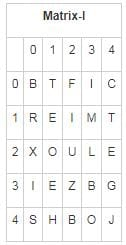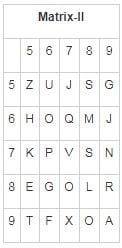Detailed Solution for RRB NTPC Mock Test - 11 (English) - Question 58

1) 41, 56, 10, 95 → H, U, R, T

2) 65, 56, 87, 13 → H, U, O, M

3) 65, 12, 89, 01 → H, I, R, T

4) 41, 22, 87, 01 → H, U, O, T

Hence, 41, 56, 10, 95 is the correct answer.

RRB NTPC Mock Test - 11 (English) - Question 59

Which of the following is not an example of Allotrope?

Detailed Solution for RRB NTPC Mock Test - 11 (English) - Question 59

Allotropes are generally elements which exist in two or more different forms in the same physical state and they also differ in physical properties and chemical activity. Diamond, graphite, and fullerenes are three allotropes of the element carbon. Steel, on the other hand, is an alloy of iron and carbon and other elements.

RRB NTPC Mock Test - 11 (English) - Question 60

Find out the correct answer for the unsolved equation based on a certain system.

32 + 64 = 15, 84 + 18 = 21, 93 + 24 = ?

Detailed Solution for RRB NTPC Mock Test - 11 (English) - Question 60

(3 + 2) + (6 + 4) = 5 + 10 = 15,

(8 + 4) + (1 + 8) = 12 + 9 = 21,

Similarly,

(9 + 3) + (2 + 4) = 12 + 6 = 18.

So, the missing term is 18.

RRB NTPC Mock Test - 11 (English) - Question 61

Which of the following jumble word is the name of an Indian River?

Detailed Solution for RRB NTPC Mock Test - 11 (English) - Question 61

1) ANGAG = GANGA (INDIA)

2) ILNE = NILE (NORTH AFRICA)

3) NAZAMO = AMAZON (SOUTH AMERICA)

4) ONGOC = CONGO (AFRICA)

Hence option ANGAG is the correct answer.

RRB NTPC Mock Test - 11 (English) - Question 62

Who became the first Asian woman cricketer to play 100 T20 international matches as on 4 February 2019?

Detailed Solution for RRB NTPC Mock Test - 11 (English) - Question 62
• Sana Mir from Pakistan became the first Asian woman cricketer to play 100 T20 international matches.
• She achieved the milestone during the final T20 against West Indies in Karachi.
• Overall, she is the 6th woman cricketer to complete a century of T20 internationals. Daendra Dottin from West Indies ranks first with a total of 109 T20s to her name.
RRB NTPC Mock Test - 11 (English) - Question 63

Which musical instrument Ustad Bismillah Khan used to play?

Detailed Solution for RRB NTPC Mock Test - 11 (English) - Question 63
• Ustad Bismillah Khan used to play Shehnai.
• Ustad Bismillah Khan (1916 – 2006) (Real name is Qamaruddin Khan), was an Indian musician credited with popularizing the Shehnai.
• Shehnai is a musical instrument which is similar to the oboe (common in India, Pakistan, and Bangladesh.)
• He was honoured with India's highest civilian honour, the Bharat Ratna in 2001 thus becoming the third classical musician after M. S. Subbulakshmi and Ravi Shankar to be awarded this distinction.
• On his 102nd birth anniversary, he was honoured by Google with a Google doodle.
RRB NTPC Mock Test - 11 (English) - Question 64

Where has the 34th edition of AAHAR - the International Food and Hospitality fair - begun on 12 March 2019?

Detailed Solution for RRB NTPC Mock Test - 11 (English) - Question 64
• The 34th edition of AAHAR - the International Food and Hospitality fair - began in New Delhi on 12 March 2019.
• The fair will feature a wide range of food products, machinery, hospitality and Decoration and Confectionery items from over 560 participants from India and abroad.
• Around 20 countries, including the US, Russia, China, UK, and Germany will participate in the 5-day fair.
RRB NTPC Mock Test - 11 (English) - Question 65

Who among the following was the founder of Indian Reform Association?

Detailed Solution for RRB NTPC Mock Test - 11 (English) - Question 65
• Keshab Chandra Sen was the founder and first president of Indian Reform Association.
• It was formed on 29 October 1870 at Calcutta.
• The main objective of this organisation was to create opinion against child marriages and for legalising the Brahmo form of marriage.
RRB NTPC Mock Test - 11 (English) - Question 66

In the question, a statement is given, followed by two assumptions, I and II. You have to consider the statement to be true even if it seems to be at variance from commonly known facts. You have to decide which of the given assumption is implicit in the statement.

Statement: John's recent investment in stock market is a gamble.

Assumption I: He may gain from his investment
Assumption II: He may incur a loss from his investment.

Detailed Solution for RRB NTPC Mock Test - 11 (English) - Question 66

Usage of the word gamble denotes that he may either win or lose due to his investment. This makes either assumption I or assumption II implicit.

RRB NTPC Mock Test - 11 (English) - Question 67

A piece of paper is folded and punched as shown below in the question figures. From the given answer figures, indicate how it will appear when opened?Detailed Solution for RRB NTPC Mock Test - 11 (English) - Question 67Hence, option 3) is the correct answer figure.

RRB NTPC Mock Test - 11 (English) - Question 68

Which among the following sectors of Economy has seen a maximum rise since economic reforms in India?

Detailed Solution for RRB NTPC Mock Test - 11 (English) - Question 68

The tertiary or the service sector of the economy has seen a maximum rise since economic reforms in India as compared to other services.

RRB NTPC Mock Test - 11 (English) - Question 69

Simplify :
95 - [144 ÷ (12 × 12) - (-4) - {3 – 17 - 10}]

Detailed Solution for RRB NTPC Mock Test - 11 (English) - Question 69

95 - [144 ÷ (12 × 12) - (-4) - {3 - 17 - 10}]

= 95 - [144 ÷ (12 × 12) - (-4) - {3 - 27}]

= 95 - [144 ÷ 144 - (-4) - {-24)]

= 95 - [1 - (-4) - (-24)]

= 95 - [1 + 4 + 24]

= 95 - 29

= 66

RRB NTPC Mock Test - 11 (English) - Question 70

The ratio of present ages of A and B is 2 : 3. If the age of B after 5 years will be 20 years, then what is the present age (in years) of A?

Detailed Solution for RRB NTPC Mock Test - 11 (English) - Question 70

Let present ages of A and B be 2k and 3k

Five years later the age of B will be 20 years

Then;

(3k + 5) = 20

→ 3k = 20 - 5

→ 3k = 15

→ k = 15/3

k = 5

Hence, present age of A is 2 × 5 = 10 years.

RRB NTPC Mock Test - 11 (English) - Question 71

Who among the following is related with 'Labour Theory of Property'?

Detailed Solution for RRB NTPC Mock Test - 11 (English) - Question 71
• Labour Theory of Property was given by John Locke.
• It states that property comes about by exertion of labour upon natural resources.
• According to Locke, when a person works on a field, that labour enters into the object thus, the object becomes the property of person.
RRB NTPC Mock Test - 11 (English) - Question 72

Find out which of the figures (1), (2), (3) and (4) can be formed from the pieces given in figure X? (using all pieces once)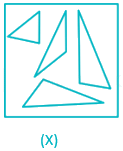Detailed Solution for RRB NTPC Mock Test - 11 (English) - Question 72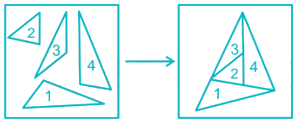Hence, the below figure can be formed from the pieces given in the figure (X).RRB NTPC Mock Test - 11 (English) - Question 73

If mirror image shows 18:18 in a 24 hr clock, then what will be the actual timing?

Detailed Solution for RRB NTPC Mock Test - 11 (English) - Question 73

For every mirror image based on a clock,

If it is 12 hrs clock, then subtract the given timing from 11:60 hrs.

If it is 24 hrs clock, then subtract the given timing from 23:60 hrs.

Here it is 24 hrs clock, therefore, 23:60 – 18:18 = 5:42

Hence, actual time will be 5:42

RRB NTPC Mock Test - 11 (English) - Question 74

A train is travelling with a speed of 64 kmph, passes a signal post in 18 s. What is the length of the train?

Detailed Solution for RRB NTPC Mock Test - 11 (English) - Question 74

Speed of train = 64kmph = 64 × 5/18 = 160/9 m/s

Time taken to pass a signal post = 18s

Length of train = Distance travelled = Speed of train × Time taken = 160/9 × 18 = 320m

Thus, length of the train = 320 m

RRB NTPC Mock Test - 11 (English) - Question 75

Who commanded the Maratha Army in the Third Battle of Panipat?

Detailed Solution for RRB NTPC Mock Test - 11 (English) - Question 75

Sadashiv Rao Bhau was commander-in-chief of the Maratha Army in the Third Battle of Panipat. The Third Battle of Panipat took place in 1761 between Ahmad Shah Abdali and Marathas.

RRB NTPC Mock Test - 11 (English) - Question 76

A man is running beside an 80 m long train at a speed of 9 km/hr in the same direction. If the train is running at a speed of 54 km/hr, in how much time will the train cross the running man?

Detailed Solution for RRB NTPC Mock Test - 11 (English) - Question 76

Speed of train relative to running man = speed of train – speed of running man

⇒ Speed of train relative to running = 54 – 9 = 45 km/hr = 45 × (5/18) = 12.5 m/sec

Distance to be covered = 80 m

∴ Time taken by train to cross the running man = 80/12.5 = 6.4 sec.

RRB NTPC Mock Test - 11 (English) - Question 77

In a certain code language, "ROAD" is written as "TREI". How is "SIGN" written in that code language?

Detailed Solution for RRB NTPC Mock Test - 11 (English) - Question 77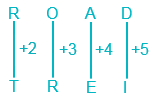Similarly,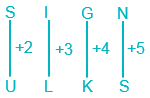Hence, ULKS is the correct answer.

RRB NTPC Mock Test - 11 (English) - Question 78

Which of the following is related to the Second Schedule of Indian Constitution?

Detailed Solution for RRB NTPC Mock Test - 11 (English) - Question 78
• Second schedule lists the emoluments for holders of constitutional offices such as salaries of President, Vice President, Ministers, Judges and Comptroller and Auditor-General of India etc.
• Representation in the RS is defined in the Fourth Schedule whereas Languages are defined in the Eighth Schedule.
• The aspects of oath taking have been taken care of in the Third Schedule of the Constitution.
RRB NTPC Mock Test - 11 (English) - Question 79

Given f(x) = 5x3 + 14x2 – 10x + 9. Given the divisor is (x + 1), what should be added or subtracted from f(x) so as to make it exactly divisible by the divisor?

Detailed Solution for RRB NTPC Mock Test - 11 (English) - Question 79

Given, f(x) = 5x3 + 14x2 – 10x + 9 and g(x) = x + 1

Now, we know,

f(x) = g(x) × q(x) + r(x)

⇒ f(x) – r(x) = g(x) × q(x)

∴ Remainder r(x) should be subtracted from f(x) so that it is exactly divisible by g(x) by a factor of q(x).

Now we divide f(x) by g(x) to obtain r(x).

5x2 + 9x – 19

⇒         x + 1 )) 5x3 + 14x2 – 10x + 9

5x3 + 5x2

9x2 – 10x + 9

9x2 + 9x

– 19x + 9

– 19x – 19

+28

∴ r(x) = 28

∴ 28 should be subtracted from f(x).

RRB NTPC Mock Test - 11 (English) - Question 80

Which type of lens is found in the human eye?

Detailed Solution for RRB NTPC Mock Test - 11 (English) - Question 80

The eye has crystalline or convex lens. It can adjust its focus and provides us with a clear picture of the objects that we see.

RRB NTPC Mock Test - 11 (English) - Question 81

4 bells ring at intervals of 30 minutes, 1 hour,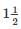hour and 1 hour 45 minutes respectively. All the bells ring simultaneously at 12 noon. They will again ring simultaneously at:

Detailed Solution for RRB NTPC Mock Test - 11 (English) - Question 81

Given data-

4 bells ring at intervals of 30 minutes, 1 hour,hour and 1 hour 45 minutes respectively

Converting them into fractions

30min = 1/2 hour

1 hour = 1/1 hourHour = 3/2 hour

1 hour 45 minutes = 7/4 hour

To find next simultaneous ring of 4 bells

Let’s find L.C.M. of these fractions:

L.C.M of fractions = (L.C.M of numerators)/(H.C.F of denominators)

⇒ LCM of fractions =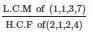⇒ LCM of fractions = 21/1 = 21

∴ Bells will ring simultaneously after 21 hours.

Counting 21 hours after 12 noon.

They will ring on 9 am. On next day.

RRB NTPC Mock Test - 11 (English) - Question 82

Mohan is Ritu's father's only son. How is Ritu related to Mohan.

Detailed Solution for RRB NTPC Mock Test - 11 (English) - Question 82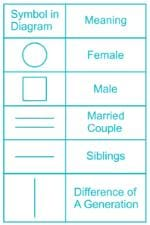According to the information,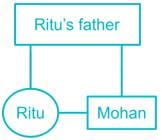Thus, Ritu is sister of Mohan.

RRB NTPC Mock Test - 11 (English) - Question 83

Who has become the first pontiff to visit UAE on 4th February 2019?

Detailed Solution for RRB NTPC Mock Test - 11 (English) - Question 83
• Pope Francis made his historic visit to UAE on 4th February 2019.
• Francis attended an inter-religious meeting and held Mass at Zayed Sports City.
• Pope Francis became the first pontiff to visit Arabian Gulf.
• His visit to UAE has highlighted religious freedom.
RRB NTPC Mock Test - 11 (English) - Question 84

What is the unit of relative density?

Detailed Solution for RRB NTPC Mock Test - 11 (English) - Question 84

Relative density = Density of the substance/Density of water at 4°C. As both the numerator and the denominator on the RHS of the above formula has the same unit (i.e. kg/m3) they get cancelled.

RRB NTPC Mock Test - 11 (English) - Question 85

There are eight persons P, Q, R, S, T, U, V and W. They score different marks in a test. P scored more marks than six persons while U scored less marks than six persons. V scored the lowest marks. R scored one mark less than P while Q and S scored 5 and 10 marks less than P respectively. T is not the highest scorer.

Q. Who was highest scorer?

Detailed Solution for RRB NTPC Mock Test - 11 (English) - Question 85

1) P scored more marks than six persons while U scored less marks than six persons.

…. > P > …. > ….. > ….. > ….. > U > …..

2) V scored the lowest marks.

…. > P > …. > ….. > ….. > ….. > U > V

3) R scored one mark less than P while Q and S scored 5 and 10 marks less than P respectively.

…. > P > R > Q > S > ….. > U > V

4) T is not the highest scorer.

(Therefore, W is the highest scorer as only W is left)

W > P > R > Q > S > T > U > V

Hence, W is the highest scorer.

RRB NTPC Mock Test - 11 (English) - Question 86

Statements followed by some conclusions are given below.

Statement:
In a one day cricket match, the total runs made by a team were 200. Out of these 120 runs were made by spinners.

Conclusions:
I. 60% of the team consists of spinners.
II. The opening batsmen were spinners.

Detailed Solution for RRB NTPC Mock Test - 11 (English) - Question 86

According to the statement, 60% of the total runs were made by spinners. But it doesn’t mean that 60% of the team consists of spinners. So, conclusion I doesn’t follow. Nothing about the opening batsmen is mentioned in the statement. So, conclusion II also does not follow.

Hence, neither I nor II follows.

RRB NTPC Mock Test - 11 (English) - Question 87

Which answer figure will complete the pattern in the following question figure?Detailed Solution for RRB NTPC Mock Test - 11 (English) - Question 87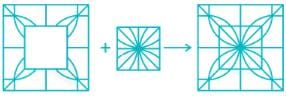Hence, figure 4 is the correct answer.

RRB NTPC Mock Test - 11 (English) - Question 88

Which of the following key combinations is used to open Windows Task Manager?

Detailed Solution for RRB NTPC Mock Test - 11 (English) - Question 88
• Ctrl + Shift + Esc are used to open Windows Task Manager.
• It is a task manager, system monitor, and startup manager which is included with Microsoft Windows systems.
RRB NTPC Mock Test - 11 (English) - Question 89

In a certain code language, "ADD" is written as "8" and "SUB" is written as "41". How is "MUL" written in that code language?

Detailed Solution for RRB NTPC Mock Test - 11 (English) - Question 89

Here, if A is 1, B is 2, C is 3 and so on Z will be 26.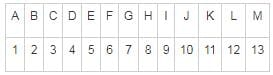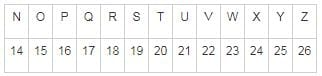The code used here is one less than the total sum of the numbers which comes in place of each letter i.e.

ADD → A = 1, D = 4, D = 4 → (1 + 4 + 4) – 1 = 9 – 1 = 8

SUB → S = 19, U = 21, B = 2 → (19 + 21 + 2) – 1 = 42 – 1 = 41

Similarly,

MUL → M = 13, U = 21, L = 12 → (13 + 21 + 12) – 1 = 46 – 1 = 45

Thus, 45 is the correct alternative.

RRB NTPC Mock Test - 11 (English) - Question 90

The Revolt of 1857 was described as 'A National Rising' by ________.

Detailed Solution for RRB NTPC Mock Test - 11 (English) - Question 90
• A leader of a Conservative Party, Benjamin Disraeli remarked the Revolt of 1857 as a national rising.
• He was a British statesman, who not only once, but served as a Prime Minister of United Kingdom, twice.
• Disraeli was born in London and was a well-known figure who was famous for his stiff policies.
RRB NTPC Mock Test - 11 (English) - Question 91

If saving exceeds investment, the national income will ________.

Detailed Solution for RRB NTPC Mock Test - 11 (English) - Question 91
• If saving exceeds investment, the national income will remain constant.
• National income is the total money earned by a country during a given year.
• When saving exceeds investment, this implies that neither money is being gained nor lost, therefore, the national income remains unaffected.
RRB NTPC Mock Test - 11 (English) - Question 92

Indra Nooyi has recently joined Amazon’s board of directors. Earlier, she was the CEO of ________.

Detailed Solution for RRB NTPC Mock Test - 11 (English) - Question 92
• PepsiCo’s India-born former CEO Indra Nooyi joined Amazon’s board of directors.
• She was Pepsico CEO from October 2006 to October 2018
• Rosalind Brewer, Starbucks Executive joined the Amazon Board earlier in February 2019.
RRB NTPC Mock Test - 11 (English) - Question 93

Who was the founder of Rashtrakuta dynasty?

Detailed Solution for RRB NTPC Mock Test - 11 (English) - Question 93

Dantidurga was the founder of the Rashtrakuta dynasty. Rashtrakuta was a royal dynasty that ruled between the sixth and the tenth century in large parts of Indian subcontinent.

RRB NTPC Mock Test - 11 (English) - Question 94

An object is sold at a price of Rs. 465 after allowing a discount of 38%. If the same object was sold at a discount for of 20%, what would be the new S.P.?

Detailed Solution for RRB NTPC Mock Test - 11 (English) - Question 94

Selling price with discount 38% is Rs. 465.

Let x be the marked price.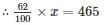New selling price =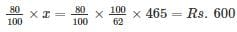RRB NTPC Mock Test - 11 (English) - Question 95

Below are given statements followed by some conclusions. You have to take the given statements to be true even if they seem to be at variance with the commonly known facts and then decide which of the given conclusions logically follow(s) from the given statements.

Statement:
Fortune favours the brave.

Conclusions:
I: Risks are necessary for success.
II: Cowards die many times before their death.

Detailed Solution for RRB NTPC Mock Test - 11 (English) - Question 95

Since the statement says nothing about cowards or about the necessity of risks. They are unrelated to the statement.

Hence, neither conclusion I nor II follows.

RRB NTPC Mock Test - 11 (English) - Question 96

Which one is the largest fraction among 3/5, 6/7 and 5/11?

Detailed Solution for RRB NTPC Mock Test - 11 (English) - Question 96

3/5 = 0.6

6/7 = 0.85

5/11 = 0.45

We can observe that 0.85 is largest among all.

∴ 6/7 is largest fraction among 3/5, 6/7, 5/11

RRB NTPC Mock Test - 11 (English) - Question 97

In the following question, select the related word pair from the given alternatives.
Tuesday : Wednesday ∷ ? : ?

Detailed Solution for RRB NTPC Mock Test - 11 (English) - Question 97

As 'Tuesday' comes before 'Wednesday',

Similarly,

‘Sunday’ comes before ‘Monday’.

Hence, Sunday : Monday is the correct answer.

RRB NTPC Mock Test - 11 (English) - Question 98

Directions: The given bar graph shows the population in the world speaking the ten most spoken languages of the world. Study the bar graph and answer the questions that follow.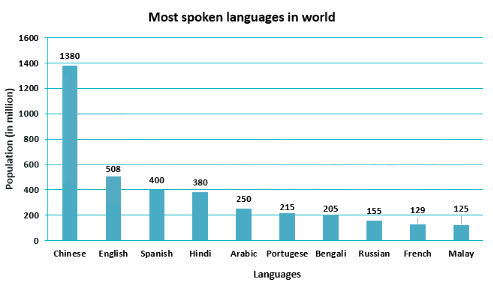Q. If the total world’s population is 7.6 billion, what percentage of the population speaks the ten most spoken languages in the world?

Detailed Solution for RRB NTPC Mock Test - 11 (English) - Question 98

Total population speaking the ten most spoken languages in the world

= 1380 + 508 + 400 + 380 + 250 + 215 + 205 + 155 + 129 + 125 = 3747 million

Total world’s population = 7.6 billion = 7.6 × 1000 = 7600 million

∴ Required percentage = (3747/7600) × 100 = 49.3%

RRB NTPC Mock Test - 11 (English) - Question 99

Directions: The given bar graph shows the population in the world speaking the ten most spoken languages of the world. Study the bar graph and answer the questions that follow.Q. If only 5.3% of the total world population speaks Spanish, then what percentage of the world’s population speaks Arabic?

Detailed Solution for RRB NTPC Mock Test - 11 (English) - Question 99

Population speaking Spanish = 400 million

Population speaking Arabic = 250 million

Let required percentage speaking Arabic be ‘x’ %

Hence,

⇒ 400/250 = 5.3/x

⇒ x = (250 × 5.3)/400

⇒ x = 3.3%

∴ 3.3% of the total world’s population speaks Arabic

RRB NTPC Mock Test - 11 (English) - Question 100

Directions: The given bar graph shows the population in the world speaking the ten most spoken languages of the world. Study the bar graph and answer the questions that follow.Q. For every 41 people speaking Bengali, how many people are there in the world that speaks Russian?

Detailed Solution for RRB NTPC Mock Test - 11 (English) - Question 100

Population speaking Bengali = 205 million

Population speaking Russian = 155 million

Let required no. of people speaking Russian be ‘x’

Hence,

⇒ 205/155 = 41/x

⇒ x = (155 × 41)/205

⇒ x = 31

∴ For every 41 people speaking Bengali, there are 31 people in the world that speaks Russian.

## RRB NTPC Mock Tests Series

20 tests
 Use Code STAYHOME200 and get INR 200 additional OFF Use Coupon Code
Information about RRB NTPC Mock Test - 11 (English) Page
In this test you can find the Exam questions for RRB NTPC Mock Test - 11 (English) solved & explained in the simplest way possible. Besides giving Questions and answers for RRB NTPC Mock Test - 11 (English), EduRev gives you an ample number of Online tests for practice

20 tests